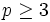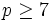# Higman's PORC conjecture

## Statement

For a prime number$p$ and a natural number$n$, define$f(p,n)$ as the number of isomorphism classes of groups of order$p^n$ (thus, the number of groups of prime power order). The conjecture is that for any fixed$n$,$f(p,n)$ is a PORC function (?) of$p$.

Note that a function on primes is PORC iff it is PORC on all but finitely many primes, because the finitely many primes can be incorporated by multiplying them with the modulus of the residue classes. Thus, the conjecture can be reformulated as stating that$f(p,n)$ is a PORC function of$p$ for all sufficiently large$p$.

## Partial truth

Higman's PORC conjecture has been verified for$n \le 7$. The PORC functions are given below.$n$ Value of PORC that works for sufficiently large$p$ Modulus relative to which we have polynomials Value of$p$ beyond which it holds Values for smaller values of$p$ Information on groups of order$p^n$
1 1 1 all$p$
2 2 1 all$p$ groups of prime-square order
3 5 1 all$p$ groups of prime-cube order
4 15 1$p \ge 3$ 14 for$p = 2$ groups of prime-fourth order
5$2p + 61 + 2\operatorname{gcd}(p-1,3) + \operatorname{gcd}(p-1,4)$ 12$p \ge 5$ 51 for$p = 2$, 67 for$p = 3$ groups of prime-fifth order
6$3p^2 + 39p + 344 + 24 \operatorname{gcd}(p - 1,3) + 11 \operatorname{gcd}(p-1,4) + 2 \operatorname{gcd}(p-1,5)$ 60$p \ge 5$ 267 for$p = 2$, 504 for$p = 3$ groups of prime-sixth order
7$3p^5 + 12p^4 + 44p^3 + 170p^2 + 707p + 2455 + (4p^2 + 44p + 291) \operatorname{gcd}(p-1, 3)$$+ (p^2 + 19p + 135) \operatorname{gcd}(p-1, 4)$$+ (3p + 31) \operatorname{gcd}(p-1, 5) + 4 \operatorname{gcd}(p-1, 7) +5 \operatorname{gcd}(p-1, 8)$$+ \operatorname{gcd}(p-1, 9)$ 360$p \ge 7$ 2328 for$p = 2$, 9310 for$p = 3$, 34297 for$p = 5$ groups of prime-seventh order

## Likely falsehood

A paper by du Sautoy and Vaughan-Lee showed that a potential refinement of the PORC conjecture that would claim that the number of immediate descendants is a PORC function turns out to be false for the order$p^{10}$ descendants of a group of order$p^9$, and explained why this meant that the PORC conjecture was likely to be false. Explicitly, what du Sautoy and Vaughan-Lee demonstrated is the following:

• In all the cases where the PORC conjecture has been established, it has been established by showing that the number of immediate descendants of a group with a prime-parametric description is a PORC function of the prime, and then summing up over a number of possibilities and using the fact that a sum of PORC functions is a PORC function.
• du Sautoy and Vaughan-Lee constructed a torsion-free nilpotent group$G$ of Hirsch length 9 and class two, arising as the du Sautoy nilpotent group for the elliptic curve$y^2 = x^3 - x$, such that the corresponding groups$G_p$ obtained by taking the quotient of$G$ by its$p^{th}$ powers for different primes$p$ behaved in a fashion that mimicked the unpredictability of the elliptic curve itself.
• They argued that one of the summands to the expression that would give the number of groups of order$p^{10}$ was not a PORC function, unlike the cases where the conjecture had been established, where all the summands are PORC functions.
• It would still be possible that there could be other summands that cancel the non-PORCness of the existing summand and still yield a sum that is a PORC function; however, the authors considered that unlikely, and believed that the sum itself would be a non-PORC function.

Thus, the result probably breaks down at$p^{10}$. Its truth for$p^8$ and$p^9$ is unknown.